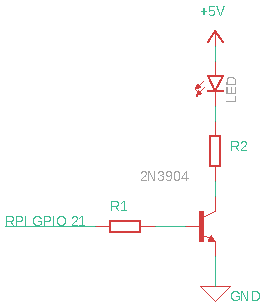2021-05-03 hardware raspberry-pi

In the previous post we introduced libgpiod. In this post we’ll use the command line tools to blink and LED. Finally, we’ll write some C code to blink the LED using the libgpiod library.

LED Driver Circuit

Skip this section if you already have a good circuit to drive an LED. Here is what I used which is a simple NPN Transistor, two resistors and the LED.Let’s say you have an LED like this. This LED can take a maximum of $30mA$, so let’s reduce to $20mA$. The LED has a Forward Voltage of $V_f=1.8V$ typical. The current going through the LED is:

$20mA = \frac{5V - 1.8V}{ R_2 }$

Solving for $R_2$ we have:

$R_2 = \frac{3.2V}{20mA} = 160\Omega$.

For $R_1$ let’s just drive $1mA$ out the GPIO Pin 21 so we have:

$1mA = \frac{3.3V - 0.7V}{R_1}$.

Solving for $R_1$ we have:

$R_1 = \frac{2.6V}{16mA} = 2600 \Omega$.

For both values $R_1$ and $R_2$ rounding down gives less current and going up risks damaging the LED and Rasberry Pi. Thus, we can use $2.7k\Omega$ for a standard value. The value of $160 \Omega$ is already standard.

You can easily plug the values for a different LED into the equations above to get the right resistor values.

We can turn the LED on using the gpioset command:

$gpioset gpiochip0 21=1 and turn it off again with:$ gpioset gpiochip0 21=0

or we can turn it on for a duration:

$gpioset --mode=time --mode=time --sec=3 gpiochip0 21=1 C Code to Blink the LED // file blink.c #include <gpiod.h> #include <error.h> #include <stdlib.h> #include <stdio.h> #include <string.h> #include <unistd.h> struct gpiod_chip *chip; struct gpiod_line_request_config config; struct gpiod_line_bulk lines; int main(int argc, char *argv[]) { unsigned int offsets; int values; int err; chip = gpiod_chip_open("/dev/gpiochip0"); if(!chip) { perror("gpiod_chip_open"); goto cleanup; } // set pin 21 to 1 (logic high) offsets = 21; values = 0; err = gpiod_chip_get_lines(chip, offsets, 1, &lines); if(err) { perror("gpiod_chip_get_lines"); goto cleanup; } memset(&config, 0, sizeof(config)); config.consumer = "blink"; config.request_type = GPIOD_LINE_REQUEST_DIRECTION_OUTPUT; config.flags = 0; // get the bulk lines setting default value to 0 err = gpiod_line_request_bulk(&lines, &config, values); if(err) { perror("gpiod_line_request_bulk"); goto cleanup; } // output value 1 to turn on the led values = 1; err = gpiod_line_set_value_bulk(&lines, values); if(err) { perror("gpiod_line_set_value_bulk"); goto cleanup; } sleep(1); // output value 0 to turn off the led values = 0; err = gpiod_line_set_value_bulk(&lines, values); if(err) { perror("gpiod_line_set_value_bulk"); goto cleanup; } cleanup: gpiod_line_release_bulk(&lines); gpiod_chip_close(chip); return EXIT_SUCCESS; } Building and Running It’s easy to build and run:$ gcc -o blink blink.o -lgpiod

You should see an LED blink for 3 seconds.